• 六次多项式的定义
万次阅读 多人点赞
2018-02-10 08:51:50

1.轨迹规划的定义
轨迹规划(trajectory planning)是运动规划(motion planning)研究的主要内容。运动规划指的是运动插补，在起始点和终止点之间插入中间点序列，实现沿着轨迹的平稳运动。运动控制包含路径规划(path planning)和轨迹规划，路径规划是规划位置，在起终点之间经过的路径点，轨迹规划是规划时间，将路径点与时间相对应。
对于我们的六轴机器人而言轨迹规划可以分为：关节空间轨迹规划和笛卡尔空间轨迹规划。关节空间轨迹规划是把机器人的关节变量变换成跟时间的函数，然后对角速度和角加速度进行约束。笛卡尔空间轨迹规划是把机器人末端在笛卡尔空间的位移、速度和加速度变换成跟时间的函数关系。
五次多项式插值能够解决三次多项式插值的角速度变化不平滑且加速度存在跳变的情况。
2.数学基础
五次多项式插值，角位移、角速度和角加速度的函数表达式为：

θ(t)=a0+a1t+a2t2+a3t3+a4t4+a5t5θ˙(t)=a1+2a2t+3a3t2+4a4t3+5a5t4θ¨(t)=2a2+6a3t+12a4t2+20a5t3 { θ ( t ) = a 0 + a 1 t + a 2 t 2 + a 3 t 3 + a 4 t 4 + a 5 t 5 θ ˙ ( t ) = a 1 + 2 a 2 t + 3 a 3 t 2 + 4 a 4 t 3 + 5 a 5 t 4 θ ¨ ( t ) = 2 a 2 + 6 a 3 t + 12 a 4 t 2 + 20 a 5 t 3

约束条件如下，相对三次多项式插值，增加了对起止点角速度的约束（为简便计算设 t0 t 0 $t_0$为0）
θ(t0)=θ0=a0θ(tf)=θf=a0+a1tf+a2t2f+a3t3f+a4t4f+a5t5fθ˙(t0)=θ˙0=a1θ˙(tf)=θ˙f=a1+2a2tf+3a3t2f+4a4t3f+5a5t4fθ¨(t0)=θ¨0=2a2θ¨(tf)=θ¨f=2a2+6a3tf+12a4t2f+20a5t3f { θ ( t 0 ) = θ 0 = a 0 θ ( t f ) = θ f = a 0 + a 1 t f + a 2 t f 2 + a 3 t f 3 + a 4 t f 4 + a 5 t f 5 θ ˙ ( t 0 ) = θ ˙ 0 = a 1 θ ˙ ( t f ) = θ ˙ f = a 1 + 2 a 2 t f + 3 a 3 t f 2 + 4 a 4 t f 3 + 5 a 5 t f 4 θ ¨ ( t 0 ) = θ ¨ 0 = 2 a 2 θ ¨ ( t f ) = θ ¨ f = 2 a 2 + 6 a 3 t f + 12 a 4 t f 2 + 20 a 5 t f 3

求解得
a0=θ0a1=θ˙0a2=θ¨02a3=20θf20θ0(8θ˙f+12θ˙0)tf(3θ¨0θ¨f)t2f2t3fa4=30θ030θf+(14θ˙f+16θ˙0)tf+(3θ¨02θ¨f)t2f2t4fa5=12θf12θ0(6θ˙f+6θ˙0)tf(θ¨0θ¨f)t2f2t5f { a 0 = θ 0 a 1 = θ ˙ 0 a 2 = θ ¨ 0 2 a 3 = 20 θ f − 20 θ 0 − ( 8 θ ˙ f + 12 θ ˙ 0 ) t f − ( 3 θ ¨ 0 − θ ¨ f ) t f 2 2 t f 3 a 4 = 30 θ 0 − 30 θ f + ( 14 θ ˙ f + 16 θ ˙ 0 ) t f + ( 3 θ ¨ 0 − 2 θ ¨ f ) t f 2 2 t f 4 a 5 = 12 θ f − 12 θ 0 − ( 6 θ ˙ f + 6 θ ˙ 0 ) t f − ( θ ¨ 0 − θ ¨ f ) t f 2 2 t f 5

3.matlab代码实现

序号位移速度加速度时间
10000
25010203
315020306
4100-15-2012
500014
clear;
clc;
q_array=[0,50,150,100,40];%指定起止位置
t_array=[0,3,6,12,14];%指定起止时间
v_array=[0,10,20,-15,0];%指定起止速度
a_array=[0,20,30,-20,0];%指定起止加速度
t=[t_array(1)];q=[q_array(1)];v=[v_array(1)];a=[a_array(1)];%初始状态
for i=1:1:length(q_array)-1;%每一段规划的时间
T=t_array(i+1)-t_array(i)
a0=q_array(i);
a1=v_array(i);
a2=a_array(i)/2;
a3=(20*q_array(i+1)-20*q_array(i)-(8*v_array(i+1)+12*v_array(i))*T-(3*a_array(i)-a_array(i+1))*T^2)/(2*T^3);
a4=(30*q_array(i)-30*q_array(i+1)+(14*v_array(i+1)+16*v_array(i))*T+(3*a_array(i)-2*a_array(i+1))*T^2)/(2*T^4);
a5=(12*q_array(i+1)-12*q_array(i)-(6*v_array(i+1)+6*v_array(i))*T-(a_array(i)-a_array(i+1))*T^2)/(2*T^5);%计算五次多项式系数
ti=t_array(i):0.001:t_array(i+1);
qi=a0+a1*(ti-t_array(i))+a2*(ti-t_array(i)).^2+a3*(ti-t_array(i)).^3+a4*(ti-t_array(i)).^4+a5*(ti-t_array(i)).^5;
vi=a1+2*a2*(ti-t_array(i))+3*a3*(ti-t_array(i)).^2+4*a4*(ti-t_array(i)).^3+5*a5*(ti-t_array(i)).^4;
ai=2*a2+6*a3*(ti-t_array(i))+12*a4*(ti-t_array(i)).^2+20*a5*(ti-t_array(i)).^3;
t=[t,ti(2:end)];q=[q,qi(2:end)];v=[v,vi(2:end)];a=[a,ai(2:end)];
end
subplot(3,1,1),plot(t,q,'r'),xlabel('t'),ylabel('position');hold on;plot(t_array,q_array,'o','color','g'),grid on;
subplot(3,1,2),plot(t,v,'b'),xlabel('t'),ylabel('velocity');hold on;plot(t_array,v_array,'*','color','y'),grid on;
subplot(3,1,3),plot(t,a,'g'),xlabel('t'),ylabel('accelerate');hold on;plot(t_array,a_array,'^','color','r'),grid on;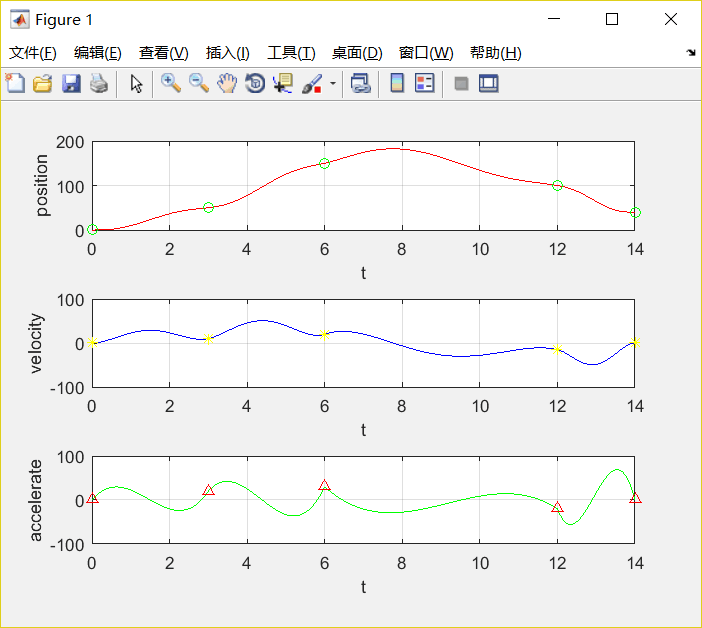PS:相对于三次多项式插值，加速度也是平滑的曲线，并没有出现跳变的情况。然而在机器人系统中，单纯的多项式规划有一个非常严重的问题：没有匀速段，无法根据期望速度提供匀速控制，而在大部分机器人应用中，对加工的速度控制都是有要求的。另一个问题就是，次数越高的多项式，加速过程越慢，整个运动过程中的平均速度越小，影响效率。下次将会为大家介绍能约束速度的三段s曲线加减速直线插值方式。

更多相关内容
• #多项式轨迹–五次多项式 1.5 Polynomial of degree five 利用三次多项式，根据过q0,q1,…,qnq_0,q_1,\ldots,q_nq0​,q1​,…,qn​确定的轨迹的特征是位置和速度连续，但是加速度不连续（参见上一篇博客轨迹规划–三...

#多项式轨迹–五次多项式

## 1.5 Polynomial of degree five

利用三次多项式，根据过 q 0 , q 1 , … , q n q_0,q_1,\ldots,q_n 确定的轨迹的特征是位置和速度连续，但是加速度不连续（参见上一篇博客轨迹规划–三次多项式轨迹）。尽管三次多项式轨迹确定的轨迹有一定“平滑”，但是对于一些应用的动力学和惯性载荷会产生一些不期待的影响。为了获得一个加速度连续的轨迹，位置和速度需要合适的初始和终止条件，也需要合适的初始和终止加速度值。这样共有六个边界条件，因此需要采用五次多项式：
q ( t ) = q 0 + a 1 ( t − t 0 ) + a 2 ( t − t 0 ) 2 + a 3 ( t − t 0 ) 3 + a 4 ( t − t 0 ) 4 + a 5 ( t − t 0 ) 5 (1-24) q(t)=q_0+a_1(t-t_0)+a_2(t-t_0)^2+a_3(t-t_0)^3+a_4(t-t_0)^4+a_5(t-t_0)^5 \tag{1-24}
根据条件
q ( t 0 ) = q 0 , q ( t 1 ) = q 1 q ˙ ( t 0 ) = v 0 , q ˙ ( t 1 ) = v 1 q ¨ ( t 0 ) = a 0 , q ¨ ( t 1 ) = a 1 . \begin{matrix} q(t_0)=q_0, &q(t_1)=q_1 \\ \dot{q}(t_0)=v_0, & \dot{q}(t_1)=v_1 \\ \ddot{q}(t_0)=\text a_0, & \ddot{q}(t_1)=\text a_1. \end{matrix}
这里定义 T = t 1 − t 0 T=t_1-t_0 ,求得多项式系数为
{ a 0 = a 0 a 1 = v 0 a 2 = 1 2 a 0 a 3 = 1 2 T 3 [ 20 h − ( 8 v 1 + 12 v 0 ) T − ( 3 a 0 − a 1 ) T 2 ] a 4 = 1 2 T 4 [ − 30 h + ( 14 v 1 + 16 v 0 ) T + ( 3 a 0 − 2 a 1 ) T 2 ] a 5 = 1 2 T 5 [ 12 h − 6 ( v 1 + v 0 ) T + ( a 1 − a 0 ) T 2 ] . (1-25) \begin{cases} a_0&=&a_0\\ a_1&=&v_0\\ a_2&=&\frac{1}{2}\text a_0\\ a_3&=&\frac{1}{2T^3}\left[20h-\left(8v_1+12v_0\right)T-(3\text a_0-\text a_1)T^2\right]\\ a_4&=&\frac{1}{2T^4}[-30h+(14v_1+16v_0)T+(3\text a_0-2\text a_1)T^2]\\ a_5&=&\frac{1}{2T^5}[12h-6(v_1+v_0)T+(\text a_1-\text a_0)T^2]. \end{cases} \tag{1-25}
Example2.9:使用五次多项式确定运动轨迹：

(a)
t 0 = 0 , t 1 = 8 , q 0 = 0 , q 1 = 10 , v 0 = 0 , v 1 = 0 , a 0 = 0 , a 1 = 0. \begin{matrix} t_0=0,& t_1=8, \\ q_0=0,& q_1=10, \\ v_0=0, &v_1=0,\\ \text a_0=0, &\text a_1=0. \end{matrix}

(b)
$$\begin{matrix} t_0&=&0,& t_1&=&8, \ q_0&=&0,& q_1&=&10, \ v_0&=&-5, &v_1&=&-10,\ \text a_0&=&0, & \text a_1&=&0. \end{matrix}$$
可以注意到，使用三次多项式是无法指定加速度的边界条件的。对(a)和(b)条件使用五次多项式确定轨迹如下图所示。可以与上一篇博文的三次多项式（轨迹规划–三次多项式轨迹）比较一下，三次多项式是无法指定加速度的边界条件的。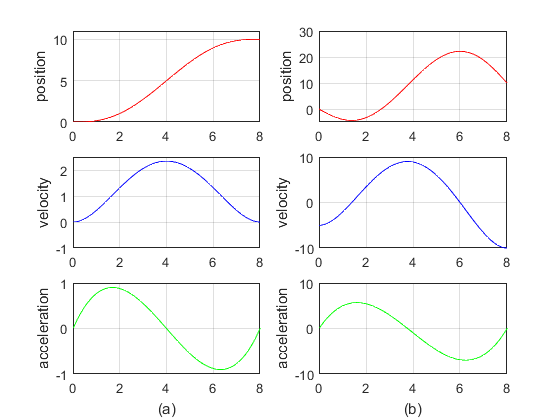图7 五次多项式轨迹

matlab仿真代码参见examplesCode文件夹下的example2_9.m文件

%example2.9
clc
clear
%轨迹定义条件
%时间
t0=0;
t1=8;
%位置和速度（a）
q0=0;
q1=10;
v0=0;
v1=0;
acc0=0;
acc1=0;
%利用公式（1-25）求系数
h=q1-q0;
T=t1-t0;
a0=q0;
a1=v0;
a2=1.0/2*acc0;
a3=1.0/(2*T*T*T)*(20*h-(8*v1+12*v0)*T+(acc1-3*acc0)/(T*T));
a4=1.0/(2*T*T*T*T)*(-30*h+(14*v1+16*v0)*T+(3*acc0-2*acc1)/(T*T));
a5=1.0/(2*T*T*T*T*T)*(12*h-6*(v1+v0)*T+(acc1-acc0)/(T*T));
%轨迹生成
t=t0:0.1:t1;
%位置
q=a0+a1*power((t-t0),1)+a2*power((t-t0),2)+a3*power((t-t0),3)+...
a4*power(t-t0,4)+a5*power(t-t0,5);
%速度
v=a1+2*a2*power((t-t0),1)+3*a3*power((t-t0),2)+4*a4*power(t-t0,3)+...
5*a5*power(t-t0,4);
%加速度
acc=2*a2+6*a3*power((t-t0),1)+12*a4*power(t-t0,2)+20*a5*power(t-t0,3);
%绘图
subplot(3,2,1)
plot(t,q,'r');
axis([0,8,0,11])
ylabel('position')
grid on
subplot(3,2,3)
plot(t,v,'b');
axis([0,8,-1,2.5])
ylabel('velocity')
grid on
subplot(3,2,5)
plot(t,acc,'g');
xlabel('(a)');
ylabel('acceleration')
grid on

%时间
t0=0;
t1=8;
%位置和速度（a）
q0=0;
q1=10;
v0=-5;
v1=-10;
acc0=0;
acc1=0;
%利用公式（1-25）求系数
h=q1-q0;
T=t1-t0;
a0=q0;
a1=v0;
a2=1.0/2*acc0;
a3=1.0/(2*T*T*T)*(20*h-(8*v1+12*v0)*T+(acc1-3*acc0)/(T*T));
a4=1.0/(2*T*T*T*T)*((-30*h+(14*v1+16*v0)*T)+(3*acc0-2*acc1)/(T*T));
a5=1.0/(2*T*T*T*T*T)*(12*h-6*(v1+v0)*T+(acc1-acc0)/(T*T));
%轨迹生成
t=t0:0.1:t1;
%位置
q=a0+a1*power((t-t0),1)+a2*power((t-t0),2)+a3*power((t-t0),3)+...
a4*power(t-t0,4)+a5*power(t-t0,5);
%速度
v=a1+2*a2*power((t-t0),1)+3*a3*power((t-t0),2)+4*a4*power(t-t0,3)+...
5*a5*power(t-t0,4);
%加速度
acc=2*a2+6*a3*power((t-t0),1)+12*a4*power(t-t0,2)+20*a5*power(t-t0,3);
%绘图
subplot(3,2,2)
plot(t,q,'r');
axis([0,8,-5,30])
ylabel('position')
grid on
subplot(3,2,4)
plot(t,v,'b');
ylabel('velocity')
grid on
subplot(3,2,6)
plot(t,acc,'g');
xlabel('(b)');
ylabel('acceleration')
grid on


Example 2.10:对于example2.7、example2.8 的条件（参见上一篇博客轨迹规划–三次多项式轨迹）使用五次多项式确定轨迹，如下图所示。并与example2.8对比，发现“平滑度”得到了改善。

给定条件
t 0 = 0 , t 1 = 2 , t 2 = 4 , t 3 = 8 , t 4 = 10 , q 0 = 10 , q 1 = 20 , q 2 = 0 , q 3 = 30 , q 4 = 40 , v 0 = 0 , v 1 = − 10 , v 2 = 10 , v 3 = 3 , v 4 = 0. \begin{matrix} {{t}_{0}}=0,{{t}_{1}}=2, &{{t}_{2}}=4,{{t}_{3}}=8,{{t}_{4}}=10, \\ {{q}_{0}}=10,{{q}_{1}}=20, &{{q}_{2}}=0,{{q}_{3}}=30,{{q}_{4}}=40,\\ {{v}_{0}}=0,{{v}_{1}}=-10, &{{v}_{2}}=10,{{v}_{3}}=3,{{v}_{4}}=0. \\ \end{matrix}
由此系列点确定的轨迹如下图所示。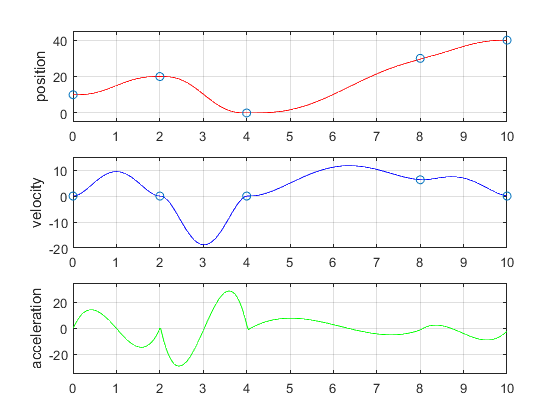图8 Example2.7的条件用五次多项式确定的轨迹

matlab仿真代码参见examplesCode文件夹下的example2_10.m文件

%example2.10
clc
clear
close('all')
%轨迹定义条件
%时间、位置和速度（a）
t_array=[0,2,4,8,10];
q_array=[10,20,0,30,40];
v_array=[0,-10,10,3,0];
%起点和终点加速度假设为0，中间点加速度都初始化为0，
acc_array=[0,0,0,0,0];
%计算轨迹
%初始位置
t=t_array(1);
q=q_array(1);
v=v_array(1);
v_array2=v_array;
acc=acc_array(1);

for k=1:length(t_array)-1
%按照式（1-23）式确定中间点的速度值
if(k>1)
dk1=(q_array(k)-q_array(k-1))/(t_array(k)-t_array(k-1));
dk2=(q_array(k+1)-q_array(k))/(t_array(k+1)-t_array(k));
if((dk2>=0 && dk1>=0) || (dk2<=0 && dk1<=0))
v_array2(k)=1.0/2.0*(dk1+dk2);
else
v_array2(k)=0;
end
end
end

for k=1:length(t_array)-1
%利用公式（1-25）求系数

%计算各段多项式的系数
h(k)=q_array(k+1)-q_array(k);
T(k)=t_array(k+1)-t_array(k);
a0(k)=q_array(k);
a1(k)=v_array2(k);
a2(k)=1.0/2*acc_array(k);
a3(k)=1.0/(2*T(k)*T(k)*T(k))*(20*h(k)-(8*v_array2(k+1)+12*v_array2(k))*T(k)+(acc_array(k+1)-3*acc_array(k))/(T(k)*T(k)));
a4(k)=1.0/(2*T(k)*T(k)*T(k)*T(k))*(-30*h(k)+(14*v_array2(k+1)+16*v_array2(k))*T(k)+(3*acc_array(k)-2*acc_array(k+1))/(T(k)*T(k)));
a5(k)=1.0/(2*T(k)*T(k)*T(k)*T(k)*T(k))*(12*h(k)-6*(v_array2(k+1)+v_array2(k))*T(k)+(acc_array(k+1)-acc_array(k))/(T(k)*T(k)));

%生成各段轨迹密化的数据点
%局部时间坐标
tau=t_array(k):T(k)/100:t_array(k+1);
%全局时间坐标，由局部时间坐标组成
t=[t,tau(2:end)];
%位置
qk=a0(k)+a1(k)*power(tau-tau(k),1)+a2(k)*power(tau-tau(k),2)+a3(k)*power(tau-tau(k),3)+...
a4(k)*power(tau-tau(k),4)+a5(k)*power(tau-tau(k),5);
%全局位置坐标
q=[q,qk(2:end)];
%速度
vk=a1(k)+2*a2(k)*power(tau-tau(k),1)+3*a3(k)*power(tau-tau(k),2)+4*a4(k)*power(tau-tau(k),3)+...
5*a5(k)*power(tau-tau(k),4);
v=[v,vk(2:end)];
%加速度
acck=2*a2(k)+6*a3(k)*power(tau-tau(k),1)+12*a4(k)*power(tau-tau(k),2)+20*a5(k)*power(tau-tau(k),3);
acc=[acc,acck(2:end)];

end
%绘图
subplot(3,1,1)
plot(t,q,'r');
hold on
plot(t_array,q_array,'o');
axis([0,10,-5,45])
ylabel('position')
grid on
subplot(3,1,2)
plot(t,v,'b');
hold on
plot(t_array,v_array2,'o');
axis([0,10,-20,15])
ylabel('velocity')
grid on
subplot(3,1,3)
plot(t,acc,'g');
axis([0,10,-35,35])
ylabel('acceleration')
grid on


接下来将介绍七次多项式和更高阶次的多项式轨迹。

参考文献

Biagiotti L, Melchiorri C. Trajectory Planning for Automatic Machines and Robots[M]. Springer Berlin Heidelberg, 2009.

展开全文多项式轨迹
• 轴机械臂本体由6个可重组的关节模组、连接部件、底座、末端部件组成，如下图所示，定义底部为第一关节（Jiont 1，其它关节命名同上）。 机械臂的参数如表2.1所示。机械臂的尺寸如图2.2所示。 ...

六轴机械臂本体由6个可重组的关节模组、连接部件、底座、末端部件组成，如下图所示，定义底部为第一关节（Jiont 1，其它关节命名同上）。
机械臂的参数如表2.1所示。机械臂的尺寸如图2.2所示。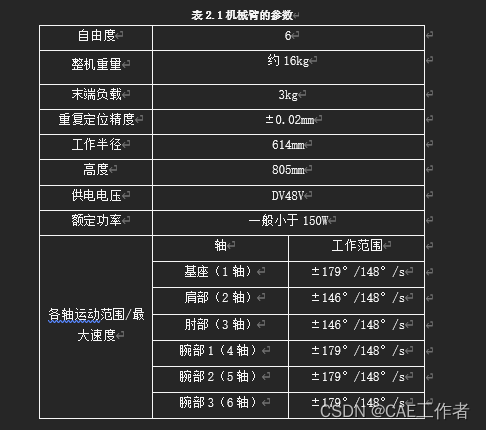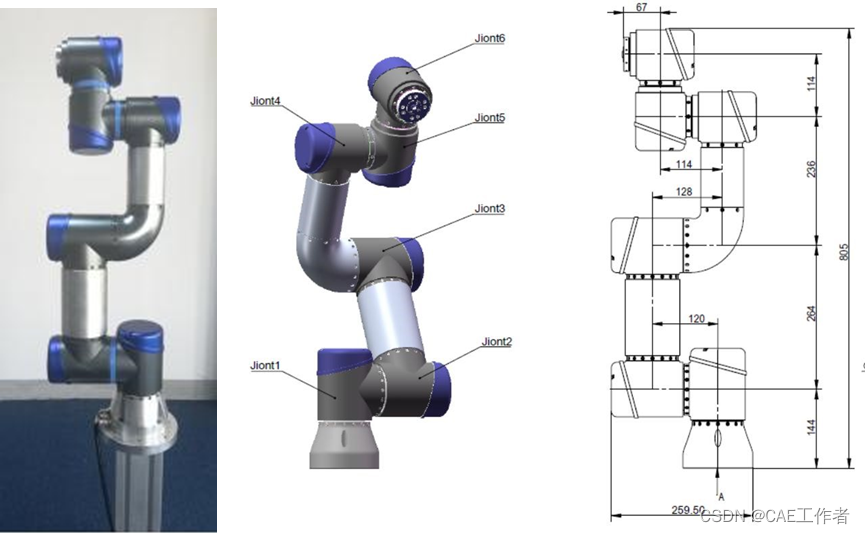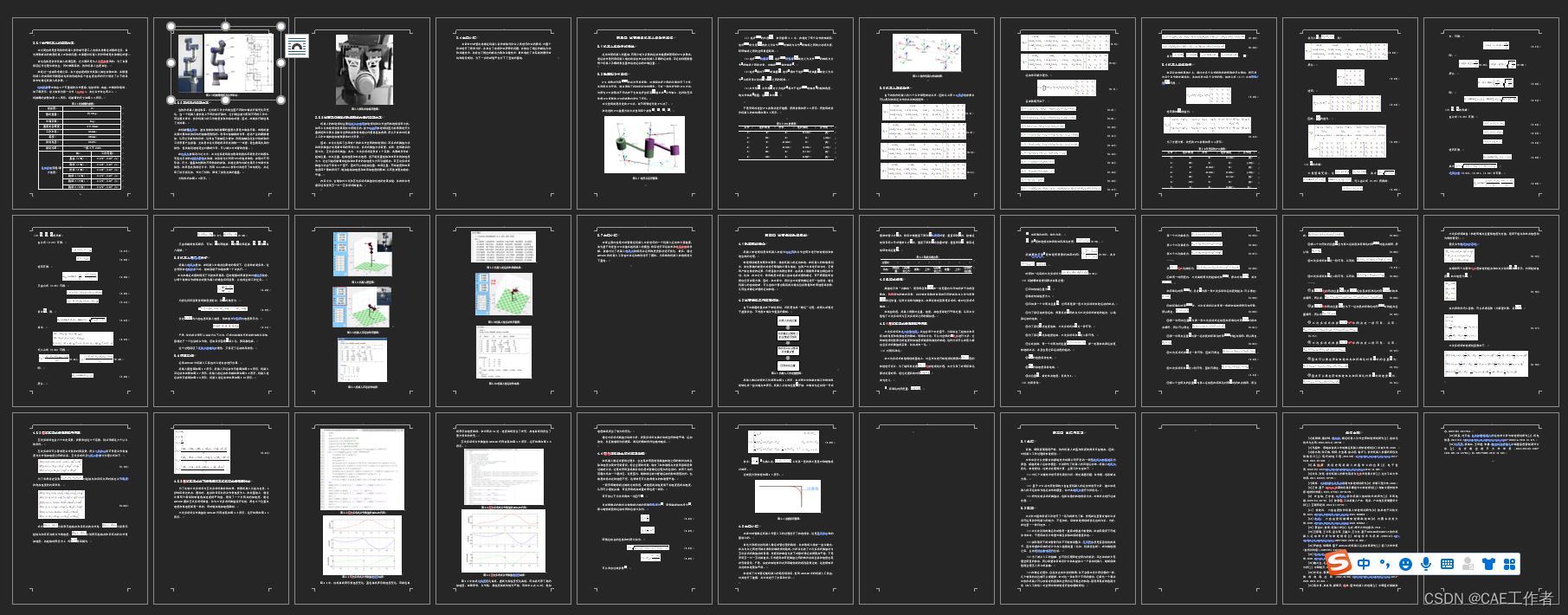展开全文学习
• 1.轨迹规划的定义 轨迹规划(trajectory planning)是运动规划(motion planning)研究的主要内容。运动规划指的是运动插补，在起始点和终止点之间插入中间点序列，实现沿着轨迹的平稳运动。运动控制包含路径规划(path ...

1.轨迹规划的定义
轨迹规划(trajectory planning)是运动规划(motion planning)研究的主要内容。运动规划指的是运动插补，在起始点和终止点之间插入中间点序列，实现沿着轨迹的平稳运动。运动控制包含路径规划(path planning)和轨迹规划，路径规划是规划位置，在起终点之间经过的路径点，轨迹规划是规划时间，将路径点与时间相对应。
对于我们的六轴机器人而言轨迹规划可以分为：关节空间轨迹规划和笛卡尔空间轨迹规划。关节空间轨迹规划是把机器人的关节变量变换成跟时间的函数，然后对角速度和角加速度进行约束。笛卡尔空间轨迹规划是把机器人末端在笛卡尔空间的位移、速度和加速度变换成跟时间的函数关系。
2.数学基础
三次多项式插值（适用于起点和终点速度为零的情况，约束关节在起点和终点的角度值，规定轨迹两端点位置角速度为定值）。
设关节角满足下式

θ(t)=a0+a1t+a2t2+a3t3θ˙(t)=a1+2a2t+3a3t2θ¨(t)=2a2+6a3t { θ ( t ) = a 0 + a 1 t + a 2 t 2 + a 3 t 3 θ ˙ ( t ) = a 1 + 2 a 2 t + 3 a 3 t 2 θ ¨ ( t ) = 2 a 2 + 6 a 3 t

将相邻两个点看作一小段轨迹的起点和终点分别用 θ0 θ 0 $\theta_0$ θf θ f $\theta_f$表示，约束起始速度为 v0 v 0 $v_0$，终止速度为 vf v f $v_f$
θ(t0)=θ0θ(tf)=θfθ˙(t0)=v0θ˙(tf)=vf { θ ( t 0 ) = θ 0 θ ( t f ) = θ f θ ˙ ( t 0 ) = v 0 θ ˙ ( t f ) = v f

将约束条件代入函数，可以求得系数（为简便计算，设 t0=0 t 0 = 0 $t_0=0$
a0=θ0a1=v0a2=3t2f(θfθ0)1tf(2v0+vf)a3=2t3f(θ0θf)+1t2f(v0+vf) { a 0 = θ 0 a 1 = v 0 a 2 = 3 t f 2 ( θ f − θ 0 ) − 1 t f ( 2 v 0 + v f ) a 3 = 2 t f 3 ( θ 0 − θ f ) + 1 t f 2 ( v 0 + v f )

三次多项式规划轨迹如下
θ(t)=θ0+v0t+[3t2f(θfθ0)1tf(2v0+vf)]t2+[2t3f(θ0θf)+1t2f(v0+vf)]t3θ˙(t)=v0+2[3t2f(θfθ0)1tf(2v0+vf)]t+3[2t3f(θ0θf)+1t2f(v0+vf)]t2θ¨(t)=2[3t2f(θfθ0)1tf(2v0+vf)]+6[2t3f(θ0θf)+1t2f(v0+vf)]t { θ ( t ) = θ 0 + v 0 t + [ 3 t f 2 ( θ f − θ 0 ) − 1 t f ( 2 v 0 + v f ) ] t 2 + [ 2 t f 3 ( θ 0 − θ f ) + 1 t f 2 ( v 0 + v f ) ] t 3 θ ˙ ( t ) = v 0 + 2 [ 3 t f 2 ( θ f − θ 0 ) − 1 t f ( 2 v 0 + v f ) ] t + 3 [ 2 t f 3 ( θ 0 − θ f ) + 1 t f 2 ( v 0 + v f ) ] t 2 θ ¨ ( t ) = 2 [ 3 t f 2 ( θ f − θ 0 ) − 1 t f ( 2 v 0 + v f ) ] + 6 [ 2 t f 3 ( θ 0 − θ f ) + 1 t f 2 ( v 0 + v f ) ] t

当速度为零时适用于一段轨迹的起终点，不为零时适用于一段轨迹的经过点。
3.matlab代码实现

序号位置速度时间
1000
210003
clear;
clc;
q0=0;
q1=100; %指定起止位置
t0=0;
t1=3;%指定起止时间
v0=0;
v1=0;%指定起止速度
a0=q0;
a1=v0;
a2=(3/(t1)^2)*(q1-q0)-(1/t1)*(2*v0+v1);
a3=(2/(t1)^3)*(q0-q1)+(1/t1^2)*(v0+v1);%计算三次多项式系数
t=t0:0.01:t1;
q=a0+a1*t+a2*t.^2+a3*t.^3;%三次多项式插值的位置
v=a1+2*a2*t+3*a3*t.^2;%三次多项式插值的速度
a=2*a2+6*a3*t;%三次多项式插值的加速度
subplot(3,1,1),plot(t,q),xlabel('t'),ylabel('position');grid on;
subplot(3,1,2),plot(t,v),xlabel('t'),ylabel('velocity');grid on;
subplot(3,1,3),plot(t,a),xlabel('t'),ylabel('accelerate');grid on;

下图为插值结果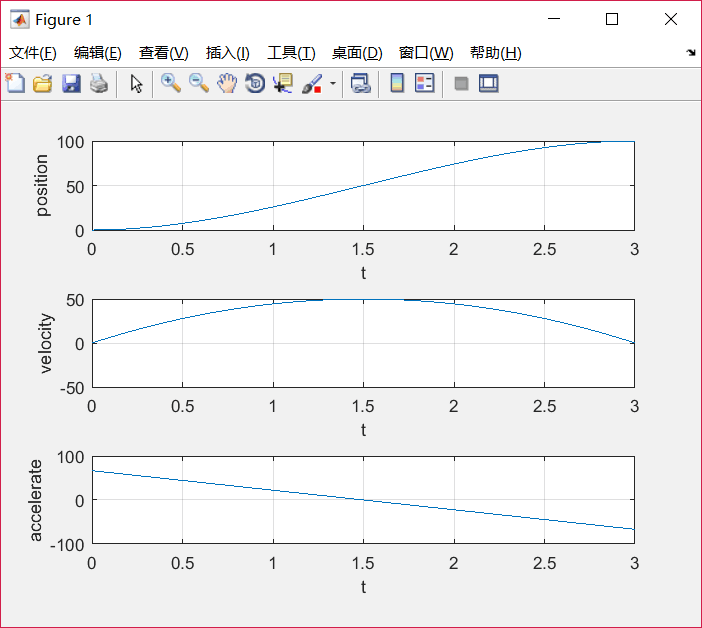4.含经过点的插值

序号位置速度时间
1000
250103
3150206
4100-1512
50014
clear;
clc;
q_array=[0,50,150,100,0];%指定起止位置
t_array=[0,2,4,8,10];%指定起止时间
v_array=[0,10,20,-15,0];%指定起止速度
t=[t_array(1)];q=[q_array(1)];v=[v_array(1)];a=;%初始状态
for i=1:1:length(q_array)-1;%每一段规划的时间
a0=q_array(i);
a1=v_array(i);
a2=(3/(t_array(i+1)-t_array(i))^2)*(q_array(i+1)-q_array(i))-(1/(t_array(i+1)-t_array(i)))*(2*v_array(i)+v_array(i+1));
a3=(2/(t_array(i+1)-t_array(i))^3)*(q_array(i)-q_array(i+1))+(1/(t_array(i+1)-t_array(i))^2)*(v_array(i)+v_array(i+1));%计算三次多项式系数
ti=t_array(i)+0.001:0.001:t_array(i+1);
qi=a0+a1*(ti-t_array(i))+a2*(ti-t_array(i)).^2+a3*(ti-t_array(i)).^3;
vi=a1+2*a2*(ti-t_array(i))+3*a3*(ti-t_array(i)).^2;
ai=2*a2+6*a3*(ti-t_array(i));
t=[t,ti];q=[q,qi];v=[v,vi];a=[a,ai];
end
subplot(3,1,1),plot(t,q,'r'),xlabel('t'),ylabel('position');grid on;
subplot(3,1,2),plot(t,v,'b'),xlabel('t'),ylabel('velocity');grid on;
subplot(3,1,3),plot(t,a,'g'),xlabel('t'),ylabel('accelerate');grid on;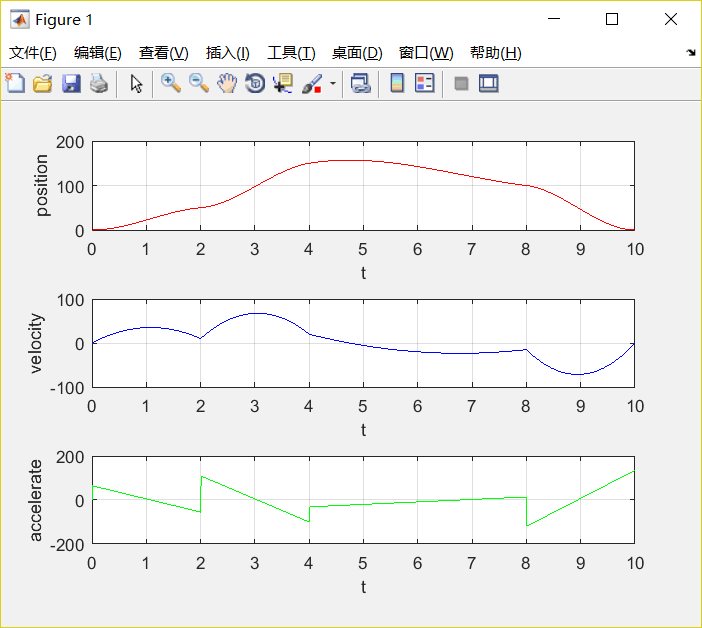PS:这种三次多项式插值，只要给定离散点位置、速度和时间，就能插补出一段连续平滑的曲线。但是角加速度并不连续，下次为大家介绍高阶多项式插值则可以解决这个问题。

展开全文插值算法
• 次多项式次多项式表达式如下： ...可以定义起始和结束的位置、速度、加速度（因为一共有个约束，所以需要是五次多项式） 举例： q0=0q_0=0q0​=0 q1=1q_1=1q1​=1 v0=0v_0=0v0​=0 v1=0v_1=机器人学
• 文章目录轨迹Joint Space下的轨迹规划三次多项式规划以矩阵的方式运算如何选择速度和加速度Example: A trajectory with one via point一般情况Example: A RRR Manipulator笛卡尔坐标系下规划关节坐标系下规划 ...动态规划
• 轨迹规划方法一：Cubic Polynomials(三次多项式) 每两个点之间用不同参数的函数来规划，找到函数把两个点串起来 任何两端相接的轨迹都要满足 smooth 的条件，也就是说每段函数头尾处的条件要定义清楚，所以一段函数...
• 轨迹规划是指机械臂在给定起始点和终止点之间运动，其中要保持时间和能量的双重最优，就需要对路径之间的插值点进行规划，目前比较常见的就是三次多项式、五次多项式以及B样条插值等进行轨迹规划。轨迹优化是指对...算法 粒子群算法 matlab
• 考虑下面的数据，虽然我们可以使用线性回归来拟合这些数据，但是这些数据更像是一条二曲线，相应的方程是y=ax2+bx+c，这个式子虽然可以理解为二方程，但是我们呢可以从另外一个角度来理解这个式子： 如果...机器学习算法
• 切比雪夫多项式切比雪夫多项式是与棣美弗定理有关，以递归方式定义的一系列正交多项式序列。通常，第一类切比雪夫多项式以符号Tn表示， 第二类切比雪夫多项式用Un表示。切比雪夫多项式 Tn 或 Un 代表 n阶多项式。...
• cubic：三次多项式插值，根据已知数据求出一个三次多项式，然后根据该多项式进行插值 %spline：三次样条插值，在每个分段区间内构造一个三次多项式，使得其插值函数满组成插值条件外，还要求在各节点处具有光滑的...
•最小二乘法 数值计算
• 1. 特征多项式定义和性质 2.特征多项式的展开（得到两个重要性质：特征值之和等于方阵的迹；特征值之积等于方阵的行列式） 3. 特征值的代数重数与几何重数的定义及其关系 4. 特征值与特征向量的...
• 实验3：取-放轨迹规划——7次多项式轨迹的规划 文章目录一、实验目的和要求1.1 目的1.2 要求二、实验手段三、轨迹规划的推导过程（7次多项式）3.1 边界条件3.2 连续条件3.3 七次多项式推导四、仿真结果与比较分析4.1...matlab仿真 PUMA560
• 次多项式插值轨迹 五次多项式插值轨迹 多项式轨迹实战 梯形速度曲线轨迹 双S形速度曲线轨迹 多个自由度轨迹的时间同步 在线轨迹规划 多项式在线轨迹规划 梯形在线轨迹规划 双S形在线轨迹规划 非线性实时轨迹...c++
• 超过七以后，能找到一个强有力的求解器机会会大大减小，TA在理论上可以求解的概率也是如此. 但是如果你的问题不是随机组成的，那么总是值得一试。算法 机器学习 人工智能
• 本章涉及知识点：1、多项式乘法的时间复杂度2、多项式的...FFT10、FFT求解多项式乘法的步骤11、python编程实战FFT大数乘法12、结果分析一、多项式乘法的时间复杂度数学中，我们可以将任意一个n位的数字写为一个n-1...
• 在数学上，一个一元n次多项式 可按降序写成： 它由n+1个系数唯一确定，因此，在计算机里他可以用一个线性表表示： 设Pn(x)和Qn(x)分别为两个一元多项式，请求出两个一元多项式的加法运算的结果，要求元素按照...数据结构
• 本文介绍回归模型的原理知识，包括线性回归、多项式回归和逻辑回归，并详细介绍Python Sklearn机器学习库的LinearRegression和LogisticRegression算法及回归分析实例。进入基础文章，希望对您有所帮助。机器学习 回归分析 线性回归
• ## 多项式朴素贝叶斯

千次阅读 2020-10-28 10:52:05
假设**X’**表示投骰子的结果， xi{x_i}xi​的取值就是[1,2,3,4,5,6]，并且这种结果是相互独立的，在样本的数量足够多的情况下，每一个点数出现的情况都是1/6。这就是多项分布。每投骰子的测试过程就是一个样本，...
• 定义如下：由若干个单项式相加组成的代数式叫做多项式（若有减法：减一个数等于加上它的相反数）。多项式中的每个单项式叫做多项式的项，这些单项式中的最高项次数，就是这个多项式的次数。其中多项式中不含字母的...算法
• 一、函数的近似表示—高次多项式 二、误差函数—最小二乘法 三、引出案例函数曲线 四、目标函数 五、优化目标函数 、优化目标函数—梯度下降法 七、优化目标函数—求解线性方程组 八、python编程实现拟合...最小二乘法
• 我们无数提到”线性“这个名词。在支持向量机中，我们也曾经提到最初的支持向量机只能够分割线性可分的数据，然后引入了”核函数“来帮助我们分类那些非线性可分的数据。我们也曾经说起过，比如说决策树，支持向量...
• ## 多项式回归

万次阅读 2018-10-31 12:24:45
1.多项式回归简介 2.scikit-learn中的多项式回归于pipeline ...考虑下面的数据，虽然我们可以使用线性回归来拟合这些数据，但是这些数据更像是一条二曲线,相应的方程是y=ax2+bx+c,这是式子虽然可...
• 多项式相乘作为一个很好的数学问题，采用编程手段会提高解决问题的效率，因此切合实际地完成程序是有很重要的意义的。计算机最开始是为了解决数学问题的数值计算而研制的，最早的编程语言如FORTRAN也是为了解决...c++...